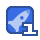# C语言程序设计报告—“万年历”.187收藏

#include"stdio.h" int judge(int year,int month)/*定义一个函数,把月分为四类(28,29,30,31)*/ { if(month==1||month==3||month==5||month==7||month==8||month==10||month==12) return(1);/*31天的月份,返回值为1*/ else if(month==2) { if(year%4!=0||year%100==0&&year%400!=0) return(2);/*平年的2月份,返回值为2*/ else return(3);/*闰年的2月份,返回值为3*/ } else return(4);/*30天的月份,返回值为4*/ } main() { int year,i,j,a,n,m,k; char ** p; char * week[]={"Sun","Mon","Tue","Wed","Thu","Fri","Sat"}; char*month[]={"January","February","March","April","May","June","July","August","September","October","November","December"}; clrscr(); printf("please inter the year:"); scanf("%d",&year); printf("\n"); printf("the calendar of the year%d.",year); printf("\n"); a=(year+(year-1)/4-(year-1)/100+(year-1)/400)%7; for(i=0;i<4;i++)/*输出前几个月的日历表*/ { n=judge(year,i+1); p=month+i; printf("%s\n",*p); printf("\n"); for(j=0;j<7;j++) {p=week+j; printf("%6s",*p);} printf("\n"); for(k=0;k<a;k++) printf(" ");/*六个空格*/ for(m=1;m<32;m++) { printf("%6d",m); if((a+m)%7==0) printf("\n");/*输出七个字符(空格和数字)换行*/ if(n==1&&m==31) break; else if(n==2&&m==28) break; else if(n==3&&m==29) break; else if(n==4&&m==30) break; } a=(a+m)%7;/*计算出下个月的第一天是星期几*/ printf("\n"); printf("============================================"); printf("\n"); } }

...展开详情

• 3
资源
• 0
粉丝
•等级C语言程序设计报告—“万年历”. 50积分/C币 立即下载
1/0